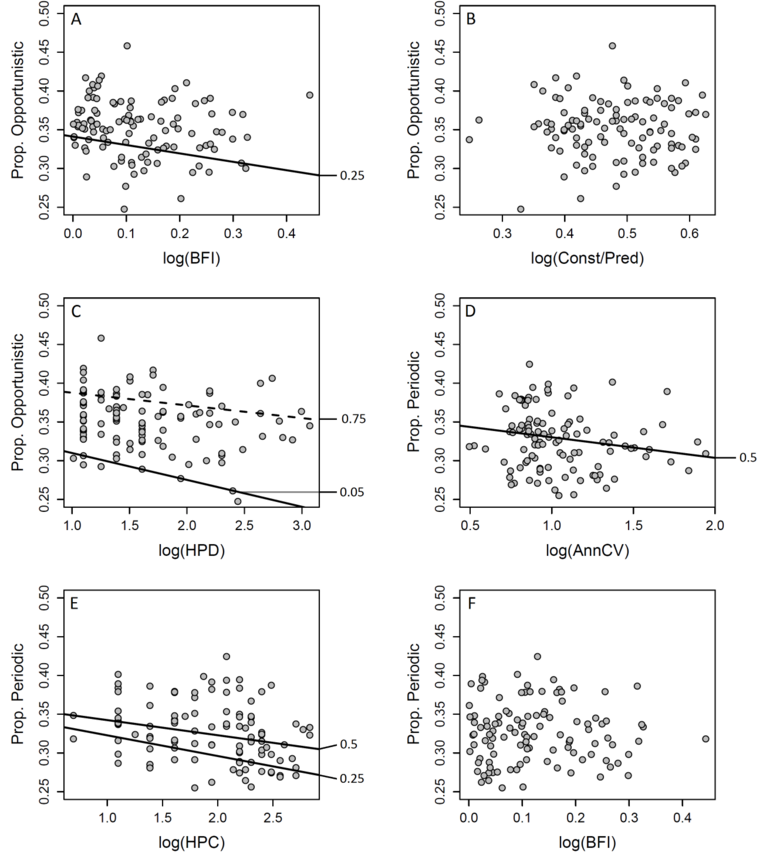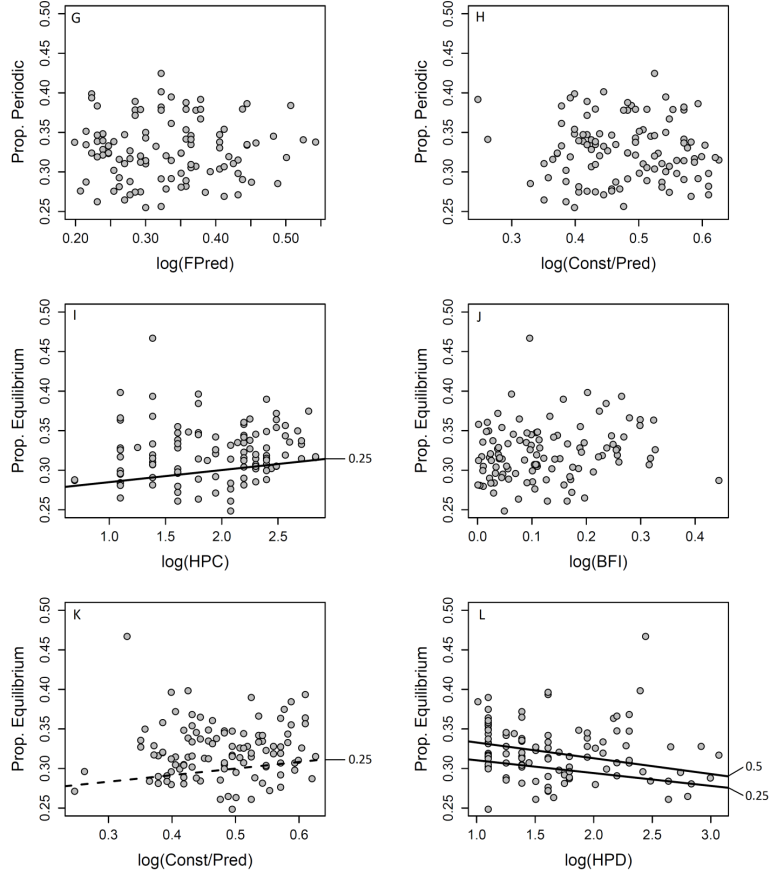Ecological Archives E093-004-A2

Meryl C. Mims and Julian D. Olden. 2012. Life history theory predicts fish assemblage response to hydrologic regimes. Ecology 93:35–45.

Appendix B. Life history and hydrologic metric relationships.

Results

Results from quantile regression analysis of relationships between hydrologic metrics and life histories that were not shown in the main text are shown in Fig. B1. To test the generality of hypothesized relationships between flow and life histories, we also examined relationships within three hydrologic classes for which sample size was sufficient: groundwater (N = 17), perennial runoff (seasonal and aseasonal combined, N = 53), and perennial flashy (seasonal and aseasonal combined, N = 33) (Table B1). Note that the perennial runoff classes and perennial flashy classes were lumped together as the ecological distinction between these classes is subtle. Extreme quantile values were set according to the guidelines of N > 5/q and N > 5/(1-q) proposed by Rogers (1992) to determine the limits of reliable extreme quantiles.

Overall, relationships between hydrologic metrics and proportional life histories across all hydrologic classes were strongest and provided the most support for a priori predictions (Fig. 4 of main text). Within hydrologic classes, relationships between metrics and proportional life histories ranged from strong correspondence with theoretical predictions but for a limited number of relationships (perennial flashy), to partially agreeing with  predictions (perennial runoff), finally to providing little support for predictions (groundwater, for which only the predicted non significant relationships were supported). Within and across hydrologic classes, a priori predictions were most supported for Opportunistic and periodic strategists, with mixed results for equilibrium strategists.

Table B1. Within flow class relationships between life histories and hydrologic indices. Relationships between proportional life history strategies and six indicators of hydrologic alteration (IHAs). Life histories include: Opportunistic, periodic, and equilibrium strategies. IHAs include: annual coefficient of variation (AnnCV), base flow index (BFI), flow predictability (FPred), high pulse count (HPC), and high pulse duration (HPD). Relationships were calculated for sites from the groundwater flow class (N = 17), perennial runoff flow class (N = 53), and perennial flashy flow class (N = 33). Relationships between proportional life history strategies and flow parameters are presented for all regression quantiles for which P ≤ 0.1 (P* ≤ 0.05). The direction of the slope of each relationship is given as positive or negative value followed by the slope direction as predicted by theory in parentheses. For cases in which the relationships observed support theoretical predictions, slope direction is underlined.

 Groundwater Relationship Model equations by quantile Regression quantile P value for slope Slope direction (predicted) Opportunistic ~ AnnCV NS NS NS NS (+) ~ HPC NS NS NS NS (+) ~ BFI NS NS NS NS (-) ~ Const/Pred NS NS NS NS (0) ~ FPred = 0.517(FPred) - 0.147 0.70 0.079 + (-) ~ HPD NS* NS NS NS (-) Periodic ~ AnnCV NS NS NS NS (-) ~ HPC NS NS NS NS (+) ~ BFI NS NS NS NS (0) ~ Const/Pred NS NS NS NS (-) ~ FPred NS NS NS NS (-) ~ HPD NS NS NS NS (-) Equilibrium ~ Ann CV = 0.080(AnnCV) + 0.258 0.30 0.027* + (-) = 0.110(AnnCV) + 0.251 0.50 0.013* + (-) = 0.092(AnnCV) + 0.274 0.70 0.035* + (-) ~ HPC = 0.020(HPC) + 0.280 0.30 0.078 + (-) ~ BFI = -0.216(BFI) + 0.383 0.30 0.001* - (+) = -0.245(BFI) + 0.430 0.70 0.038* - (+) ~ Const/Pred = -0.508(Const/Pred) + 0.615 0.30 0.072 - (+) ~ FPred = -0.455(FPred) + 0.515 0.30 0.000 - (+) = -0.636(FPred) + 0.606 0.50 0.061 - (+) = -0.497(FPred) + 0.571 0.70 0.081 - (+) ~ HPD NS NS NS NS (0) Perennial runoff Opportunistic ~ AnnCV = 0.103(AnnCV) + 0.208 0.10 0.002* + (+) = 0.077(AnnCV) + 0.256 0.25 0.086 + (+) = 0.094(AnnCV) + 0.262 0.50 0.019* + (+) = 0.116(AnnCV) + 0.261 0.75 0.000* + (+) ~ HPC = 0.039(HPC) + 0.294 0.75 0.041* + (+) ~ BFI NS NS NS NS (-) ~ Const/Pred NS NS NS NS (0) ~ FPred = -0.251(FPred) + 0.408 0.25 0.024* - (-) = -0.271(FPred) + 0.467 0.75 0.036* - (-) ~ HPD = -0.035(HPD) + 0.402 0.50 0.069 - (-) = -0.040(HPD) + 0.436 0.75 0.006* - (-) = -0.051(HPD) + 0.476 0.90 0.023* - (-) Periodic ~ AnnCV = -0.074(AnnCV) + 0.367 0.25 0.015* - (-) = -0.120(AnnCV) + 0.446 0.50 0.017* - (-) = -0.101(AnnCV) + 0.460 0.75 0.084 - (-) = -0.124(AnnCV) + 0.504 0.90 0.003* - (-) ~ HPC = -0.040(HPC) + 0.378 0.25 0.051 - (-) ~ BFI NS NS NS NS (0) ~ Const/Pred = 0.118(ConstPred) + 0.223 0.10 0.086 + (-) ~ FPred = 0.167(FPred) + 0.224 0.10 0.041* + (+) ~ HPD = 0.046(HPD) + 0.225 0.25 0.060 + (+) = 0.053(HPD) + 0.276 0.75 0.071 + (+) Equilibrium ~ AnnCV = 0.053(AnnCV) + 0.232 0.10 0.003* + (-) ~ HPC = 0.041(HPC) + 0.191 0.10 0.034* + (-) = 0.025(HPC) + 0.244 0.25 0.098 + (-) ~ BFI NS NS NS NS (+) ~ Const/Pred NS NS NS NS (+) ~ FPred NS NS NS NS (+) ~ HPD NS NS NS NS (0) Perennial flashy Opportunistic ~ AnnCV = 0.049(AnnCV) + 0.319 0.80 0.044* + (+) ~ HPC NS NS NS NS (+) ~ BFI NS NS NS NS (-) ~ Const/Pred NS NS NS NS (0) ~ FPred NS NS NS NS (-) ~ HPD NS NS NS NS (-) Periodic ~ AnnCV = -0.050(AnnCV) + 0.345 0.50 0.010* - (-) ~ HPC NS NS NS NS (-) ~ BFI NS NS NS NS (0) ~ Const/Pred NS NS NS NS (-) ~ FPred NS NS NS NS (+) ~ HPD = 0.030(HPD) + 0.305 0.80 0.091 + (+) Equilibrium ~ AnnCV NS NS NS NS (-) ~ HPC NS NS NS NS (-) ~ BFI NS NS NS NS (+) ~ Const/Pred NS NS NS NS (+) ~ Flow P NS NS NS NS (+) ~ HPD = -0.016(HPD) + 0.334 0.50 0.031* - (0) = -0.038(HPD) + 0.400 0.80 0.015* - (0)Fig. B1. Quantile regression results for relationships between hydrologic metrics (x-axis) and proportional life history composition (y-axis) with significant quantiles regression lines identified to the right of the plot (solid lines represent quantiles significant at P ≤ 0.05, and dashed lines represent quantiles significant at P ≤ 0.1). (A) Proportion of Opportunistic strategists vs. log(BFI). (B) Proportion of Opportunistic strategists vs. log(Const/Pred). (C) Proportion of Opportunistic strategists vs. log(HPD). (D) Proportion of periodic strategists vs. log(AnnCV). (E) Proportion of periodic strategists vs. log(HPC). (F) Proportion of periodic strategists vs. log(BFI). (G) Proportion of periodic strategists vs. log(FPred). (H) Proportion of periodic strategists vs. log(Const/Pred). (I) Proportion of equilibrium strategists vs. log(HPC). (J) Proportion of equilibrium strategists vs. log(BFI). (K) Proportion of equilibrium strategists vs. log(Const/Pred). (L) Proportion of equilibrium strategists vs. log(HPD).

Literature Cited

Rogers, W. H. 1992. Quantile regression standard errors. Stata Technical Bulletin 9:16–19.